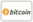Bookcover of Geometry of 2-Weierstrass points on certain plane curves
Booktitle:

# Geometry of 2-Weierstrass points on certain plane curves

## An Introduction to the geometry of higher order Weierstrass points

LAP LAMBERT Academic Publishing (2011-05-20 )eligible for voucher
ISBN-13:

### 978-3-8443-9772-7

ISBN-10:
3844397728
EAN:
9783844397727
Book language:
English
Blurb/Shorttext:
We study the 2-Weierstrass points on quartic curves. If the curve has a cyclic covering structure over P¹(ℂ), then the computation of 2-Weierstrass points is relatively easy (see Chapter 3). We deal with a 1-parameter family of smooth quartic curves without cyclic covering structures over P¹(ℂ). Let Ca be the smooth plane quartic defined by the equation: F(x,y,z)=x⁴+y⁴+z⁴+a(x²y²+x²z²+y²z²)=0, a≠-1,±2. The 1-Weierstrass points on Ca were extensively studied by Kuribayashi and his students, around 1980s. We call these quartic curves Kuribayashi quartics. In this book, we give the geometric classification of the 2-Weierstrass points on Kuribayashi quartics (see Chapter 2). In chapter 4, we study the 1-Weierstrass points on quintic curves, we see that a 1-Weierstrass point P of a smooth plane quintic C is either a flex or a sextactic point. Finally, we compute the 1-Weierstrass points on two 1-parameter families of singular plane quintics by computing special adjoint conics at these points.
Publishing house:
Website:
https://www.lap-publishing.com/
By (author) :
Alwaleed Kamel
Number of pages:
116
Published on:
2011-05-20
Stock:
Available
Category:
Arithmetic, Algebra
Price:
49.00 €
Keywords:
Algebraic Curves, Weierstrass points, Higher order Weierstrass points, quartic curves, sextactic points### Categories